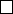4Inequalities and Lp spaces

II Probability and Measure4.2 L
p
spaces
Recall the following definition:
Definition
(Norm of vector space)
.
Let
V
be a vector space. A norm on
V
is
a function k · k : V R
0
such that
(i) ku + vk kuk + kvk for all U, v V .
(ii) kαvk = |α|kvk for all v V and α R
(iii) kvk = 0 implies v = 0.
Definition
(
L
p
spaces)
.
Let (
E, E, µ
) be a measurable space. For 1
p <
,
we define
L
p
=
L
p
(
E, E, µ
) to be the set of all measurable functions
f
such that
kfk
p
=
Z
|f|
p
dµ
1/p
< .
For p = , we let L
= L
(E, E, µ) to be the space of functions with
kfk
= inf{λ 0 : |f | λ a.e.} < .
By Minkowski’s inequality, we know
L
p
is a vector space, and also (i) holds.
By definition, (ii) holds obviously. However, (iii) does not hold for
k · k
p
, because
kfk
p
= 0 does not imply that f = 0. It merely implies that f = 0 a.e.
To fix this, we define an equivalence relation as follows: for
f, g L
p
, we say
that
f g
iff
f g
= 0 a.e. For any
f L
p
, we let [
f
] denote its equivalence
class under this relation. In other words,
[f] = {g L
p
: f g = 0 a.e.}.
Definition (L
p
space). We define
L
p
= {[f] : f L
p
},
where
[f] = {g L
p
: f g = 0 a.e.}.
This is a normed vector space under the k · k
p
norm.
One important property of
L
p
is that it is complete, i.e. every Cauchy
sequence converges.
Definition
(Complete vector space/Banach spaces)
.
A normed vector space
(
V, k · k
) is complete if every Cauchy sequence converges. In other words, if (
v
n
)
is a sequence in
V
such that
kv
n
v
m
k
0 as
n, m
, then there is some
v V
such that
kv
n
vk
0 as
n
. A complete vector space is known as
a Banach space.
Theorem.
Let 1
p
. Then
L
p
is a Banach space. In other words, if (
f
n
)
is a sequence in
L
p
, with the property that
kf
n
f
m
k
p
0 as
n, m
, then
there is some f L
p
such that kf
n
fk
p
0 as n .
Proof.
We will only give the proof for
p <
. The
p
=
case is left as an
Suppose that (
f
n
) is a sequence in
L
p
with
kf
n
f
m
k
p
0 as
n, m
.
Take a subsequence (f
n
k
) of (f
n
) with
kf
n
k+1
f
n
k
k
p
2
k
for all k N. We then find that
M
X
k=1
|f
n
k+1
f
n
k
|
p
M
X
k=1
kf
n
k+1
f
n
k
k
p
1.
We know that
M
X
k=1
|f
n
k+1
f
n
k
| %
X
k=1
|f
n
k+1
f
n
k
| as M .
So applying the monotone convergence theorem, we know that
X
k=1
|f
n
k+1
f
n
k
|
p
X
k=1
kf
n
k+1
f
n
k
k
p
1.
In particular,
X
k=1
|f
n
k+1
f
n
k
| < a.e.
So f
n
k
(x) converges a.e., since the real line is complete. So we set
f(x) =
(
lim
k→∞
f
n
k
(x) if the limit exists
0 otherwise
By an exercise on the first example sheet, this function is indeed measurable.
Then we have
kf
n
fk
p
p
= µ(|f
n
f|
p
)
= µ
lim inf
k→∞
|f
n
f
n
k
|
p
lim inf
k→∞
µ(|f
n
f
n
k
|
p
),
which tends to 0 as
n
since the sequence is Cauchy. So
f
is indeed the
limit.
Finally, we have to check that f L
p
. We have
µ(|f|
p
) = µ(|f f
n
+ f
n
|
p
)
µ((|f f
n
| + |f
n
|)
p
)
µ(2
p
(|f f
n
|
p
+ |f
n
|
p
))
= 2
p
(µ(|f f
n
|
p
) + µ(|f
n
|
p
)
2
)
We know the first term tends to 0, and in particular is finite for
n
large enough,
and the second term is also finite. So done.